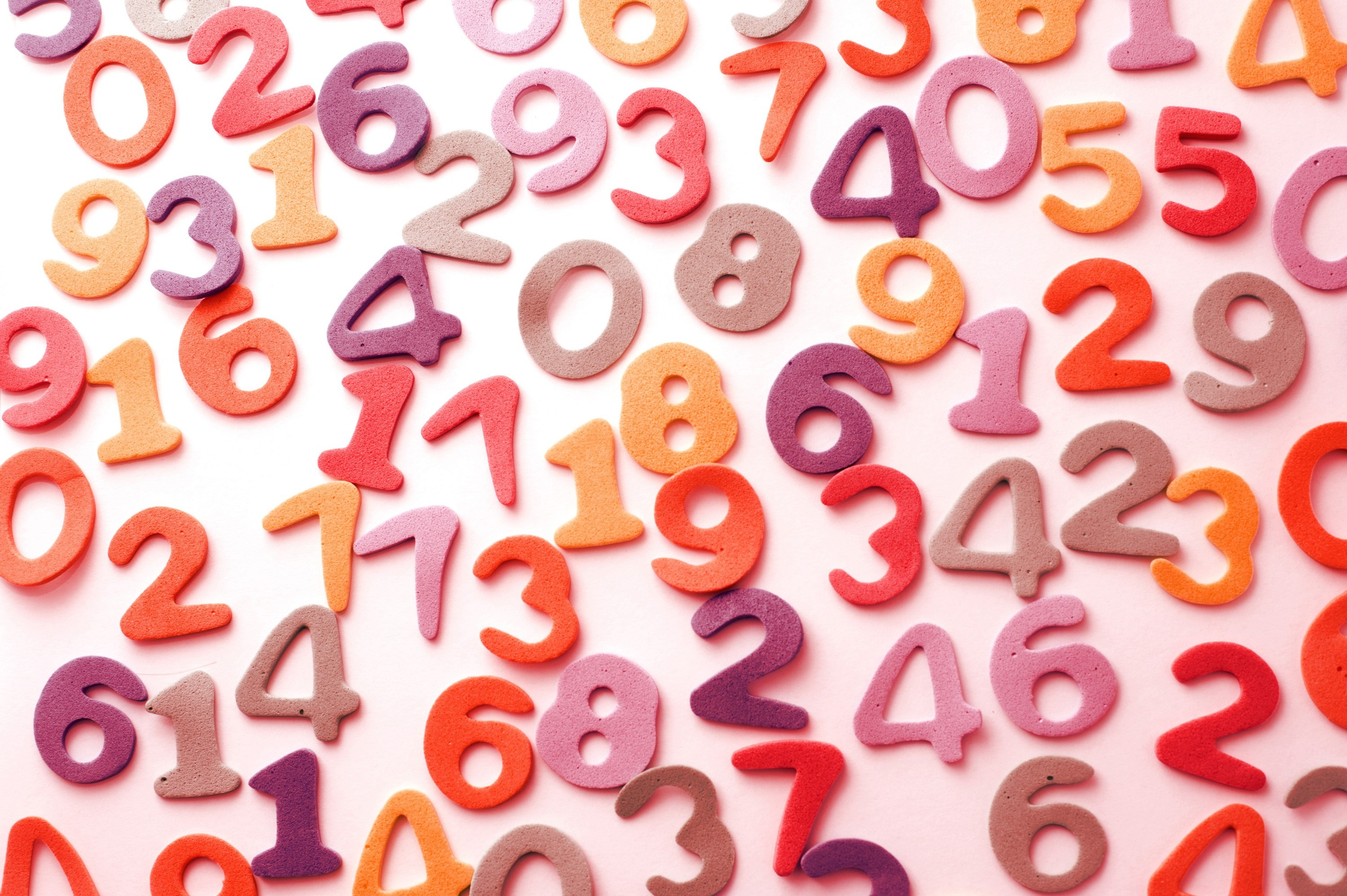1,020.8K Views

Paul, Sam and Dean are assigned the task of figuring out two numbers. They get the following information:

Both numbers are integers between (including) 1 and 1000

Both numbers may also be identical.

Paul is told the product of the two numbers, Sam the sum and Dean the difference. After receiving their number, the following conversation takes place:
Paul: I do not know the two numbers.
Sam: You did not have to tell me that, I already knew that.
Paul: Then I now know the two numbers.
Sam: I also know them.
Dean: I do not know the two numbers. I can only guess one which may probably be correct but I am not sure.
Paul: I know which one you are assuming but it is incorrect.
Dean: Ok, I also know the two numbers.

What are the two numbers?73 and 64

Here only the final statement by Paul reveals the actual numbers.

Paul is given the product

4672

and thus cannot know what the numbers are, as possible numbers are

(146, 32), (73, 64), (584, 8) and (292, 16).

However, Sam is given the sum

137, and he knows that all possible pairs are (69, 68) … (136, 1), and if given any product of these numbers, then Paul cannot deduce the factorization as there are always more than 1, thus leading to Sam stating the “I already knew that”.

But now Paul knows that the numbers must be

73 and 64, because 137 is the only sum among 146 + 32, 73 + 64, 584 + 8 and 292 + 16, of which Sam can know 100 % certainly that Paul cannot know.

Thus Paul says that he knows what the numbers are. As Paul states this, then Sam too knows the pair is

(73, 64)

because for any other numbers Paul still could not deduce the result.

Now at this point, if we did not know what numbers were given, there are only 27 possible pairs! Of these, 13 have differences that are unique, so Dean, stating that he does not know the number, means that only the 14 remaining pairings are possible:

(4, 1), (32, 23), (32, 29), (37, 16), (41, 32), (43, 16), (53, 32), (64, 37), (64, 43), (73, 64), (89, 8), (97, 16), (101, 32) and (109, 40)

There are only certain differences, and the occurrences of numbers for these differences are as follows:

3: (4, 1), (32, 29); 9: pairs (32, 23), (41, 32), (73, 64); 21: (37, 16), (53, 32), (64, 43); 27: (43, 16), (64, 37); 69: (101, 32), (109, 40); 81: (89, 8), (97, 16)

Dean must have been given 9 as the difference, as 32 is the only number appearing twice – this is the one Dean guesses as a probable number; however, Paul knows this and states that it is not in the solution, so Dean too knows that (73, 64) is the solution.